Next: Does Water Cloud the Up: Butin the End, Previous: How expensive must food

# How much will fallout tarnish saving land or nature?

By growing humanity's food on less land, farmers can surely save other land for Nature. Their accomplishment, however, may require techniques that harm the surroundings. Some would call the harm ``externalities." Others would call it ``environmental effects." I call it simply ``fallout." How much may fallout depreciate and tarnish farmers' victory of raising yields and saving land for Nature?

Farmers do some things per area, and higher yields require little more of these activities than low yields do. The land requires little more clearing, tilling, and cultivating for high yields than for low ones. Protecting a plot of lush foliage needs little more pesticide to protect it from an insect or a disease than does one of sparse foliage. Because herbicides   account for more than half of all pesticides, realizing that bumper crops require less herbicide than do sparse crops is reassuring. Dense shade as provided by bumper crops reduces the number of weeds that sprout as well as limiting the growth of the few that do. Furthermore, luxuriant foliage protects the soil from erosion better than does sparse foliage.

Later, Figure 9.3.1 will show how rising yields  increased water use   efficiency because an abundant crop consumes little more water than does one that merely shades the ground. Water is consumed more or less per area, and higher yields distill away little more water and leave little more residue of salt than do low yields.

Seed is planted per plot, and choosing a higher yielding variety has no effect on the surroundings. If the improved variety resists pests, farmers who had controlled pests with chemicals will apply less, and farmers who had suffered depredations will lose less.

On the other hand, high yields require more of certain activities than do low ones. How much will intensifying these activities or adding more of these factors per hectare affect the surroundings? Fertilizer exemplifies factors that farmers use abundantly to raise yields. I assume that less fertilizer to produce a given yield means less fallout of fertilizer into the environment or surroundings of a field.

Although the price of fertilizer and the return on it have guided past decisions, ways of adding sufficient fertilizer for abundant yields with less fallout into the environment have long been known. In fact, one scheme has lessened fertilizer use without lessening yields over an entire state (Hallberg et al., 1991[HCC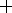91]).

In 1840, J. von Liebig virtually launched agricultural science by stating his hypothesis: raising the fertilizer element in minimum supply, the limiting factor, raises yield proportionally. In 1895, G. Liebscher, and in 1924, E. A. Mitscherlich offered amendments to Liebig's law.

Recently, deWit (1992[deW92]; 1993[deW93]) called attention to these foundations of agriculture. All three hypotheses concern a factor that limits yield and can be increased to raise yield. All state that eventually a maximum yield set by other factors places a ceiling on the increases that the limiting factor can cause. (A factor limiting yield might be, for example, fertilizer, and other factors placing a ceiling might be water and crop variety.)

What does separate the three hypotheses is the response to a limiting factor when the factor and subsequent yields are low. These responses when factor and yields are low are the initial slopes of the curves relating yield to factor in Figure 8.5.1.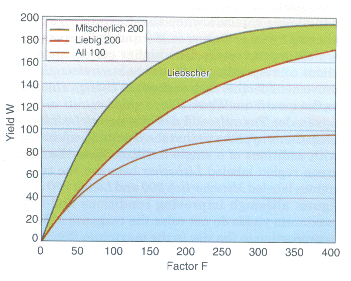Figure 8.5.1. The hypotheses of Liebig, Liebscher, and Mitscherlich, representing the rise of yield W as the limiting factor F increases. The curve All 100 shows that all three hypotheses coincide when the maximum yield Wx allowed by other factors is 100 and the response r is scaled appropriately. The higher curves show the yields corresponding to the three laws when the improvement of other factors does not change r but raises the maximum Wx to 200.

The statements about the initial slopes that differentiate the hypotheses are

• Liebig stated that whether the maximum yield is high or low a factor has one effect.
• Liebscher stated that a factor increases yield more the higher the maximum yield.
• Mitscherlich stated that a factor increases yield fully in proportion to the maximum yield.

van der Paauw (1939[vdP39]) and then deWit concluded that the results of many fertilizer trials fell between the two extremes of Liebig and Mitscherlich and so were fit by Liebscher's intermediate hypothesis.

Considering the three hypotheses as special cases of a single equation helps. The equation must show the rise of yield W as the limiting factor F increases. It must show the leveling of yield W as it approaches the maximum Wx set by other factors. And finally it must provide the range of responses to the rising Wx summarized after the bullets above. Such an equation is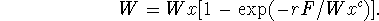The increment of yield for an increment of factor is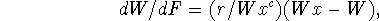which becomes the initial slope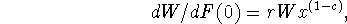when F and W are zero. When c is 1, dW/dF(0) is the constant r and represents Liebig's law.  When c is 0, dW/dF(0) is (r Wx), which is proportional to Wx and represents Mitscherlich's law.  When c is intermediate, the equation represents Liebscher's law, rising when Wx rises--but not in proportion to it.

Figure 8.5.1 shows the behavior of the three hypotheses summarized by the bullets above and by the single equation when the maximum yield is doubled from 100 to 200. The curve All 100 shows that all three hypotheses coincide when the maximum yield Wx allowed by other factors is 100 and the response r is scaled appropriately. The higher curves show the yields corresponding to the three laws when the improvement of other factors does not change r but raises the maximum Wx to 200.

van der Paauw's and deWit's surveys confirmed Liebscher's hypothesis that the results of experiments with additions of a single fertilizer factor lie in the green region labeled Liebscher between the two extremes labeled Mitscherlich 200 and Liebig 200 on the figure: although improving conditions and rising maximum yields do not raise the response of W to F in proportion to the rise in the maximum Wx, the improvement nevertheless does raise the response some, long before the limiting yield is approached.

Experiments confirm that the exponent c is neither 0 nor 1 but between those extremes. In terms of the equation, because Liebscher was correct and c is neither 0 nor 1, the response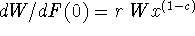clearly rises or improves as Wx improves.

I began this section by asking, ``How much may fallout depreciate saving land for Nature by raisin yields?" Altering the view of the previous figure emphasizes the answer provided by Liebscher's law. In Figure 8.5.2, I reversed axes, showing the rising amount of factor F required to produce a yield W. Remember, other things being equal, less F to produce a given W means less F to fall out. If other factors set the maximum at 100, curve All 100 shows that a yield above 100 requires an infinite amount of F and so fallout of F into the surroundings.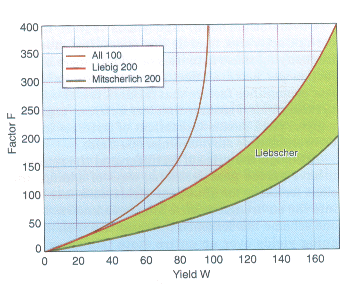Figure 8.5.2. The level of a limiting factor F required for yields W, according to the hypotheses of Liebig, Liebscher, and Mitscherlich. The curve All 100 shows that all three hypotheses coincide when the maximum yield Wx allowed by other factors is 100 and the response r is scaled appropriately. The other curves show the lesser requirements for factor F specified by the three laws when the improvement of other factors raises the maximum Wx to 200.

The crucial question, however, is whether a given, attainable yield W requires more or less F if other factors are improved. For the low yield of, say, 20, Liebig's hypothesis  states that about the same F is needed for a yield, whether other factors set a high or a low maximum. But the correct hypothesis lying in the region labeled Liebscher shows that even when yields are low, raising the maximum by improving other factors lessens the F to produce the yield and so lessens the fallout.

For the higher yield of 80, all three hypotheses show less F to produce the yield and so less F to fall out if other factors allow a maximum of 200 rather than of 100.

If other factors restrict the maximum yield to 100 a yield over 100, of course, requires an infinite amount of F and so great fallout. For yields above 100, improving other conditions and raising the maximum from 100 to 200 convincingly lowers F and its fallout.

The equation allows some reasoning about the synergism of simultaneous improvement of factors. Mitscherlich extended his equation to n factors: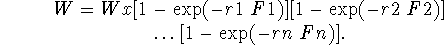When the level of the factors is low, W is approximately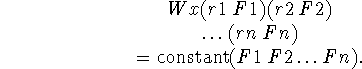If one factor Fi can be substituted by a multiple of another so that the series (F1 F2 ...Fn) can be replaced by a constant times, the approximation for W becomes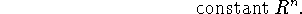and the level of R for a given yield W is approximately a constant times the nth root of R.

Clearly, doubling, tripling, and so forth all n, multiple factors now represented by R rapidly raises the initial slope or response of yield W. If there are only two factors, that is, if n = 2, doubling both factors quarters the factors needed for a given yield. Incorporating more limiting factors increases the number n of factors that will be doubled and so forth, increases the acceleration of the initial response of yield to factors, and rapidly lowers the level of the factors Fi required for a given yield W. That is the logical consequence of Mitscherlich's hypothesis.   At the other extreme, Liebig's hypothesis,  only the limiting factor F determines W. W is exactly r times F, linearly proportional to the limiting factor regardless of other factors and how they may improve Wx.

But we know that in fact the intermediate hypothesis of Liebscher's is the correct one. So I conclude that improving n factors in step lowers the level of factors needed to produce a yield somewhat more slowly than the nth root of the yield. At low levels of the factors R that are improved in step, yield is approximately R raised to the x-th power, where x lies between 1 and the number n of improved factors. Although improving n factors in step does not lower to their n-th root the level of factors needed for a yield, still it does lower the level and so the fallout.

Per area, farmers do some things about the same for high or low yields but more of other things for high yields. Clearly, raising yields to save land for Nature will not increase activities like plowing per area, nor will raising yields change such fallout as silt. In fact, reckoned per yield, such things done the same for high or low yield surely will diminish. Per ton, these things and their fallout will diminish with rising ton/hectare, just as the reciprocal hectare/ton falls. That is no surprise.

The surprise comes from the things that farmers do more of to raise yields. A high regard for the law of decreasing returns prepares us to learn that greater and greater amounts of a factor always must be applied to force ever higher yields. Instead, we have learned that removing such limitations as deficient phosphate lowers the amount of another factor like nitrogen needed to produce a given yield. Optimizing other factors raises the ceiling that causes decreasing returns and lowers the amount of a limiting factor needed to produce a given yield.

Other things being equal, the fallout   from producing the food for ten billion will be diminished as land is saved for Nature by optimizing all factors to produce more tons/hectare.Next: Does Water Cloud the Up: Butin the End, Previous: How expensive must food

Yasuko Kitajima
Thu Jun 19 16:20:56 PDT 1997